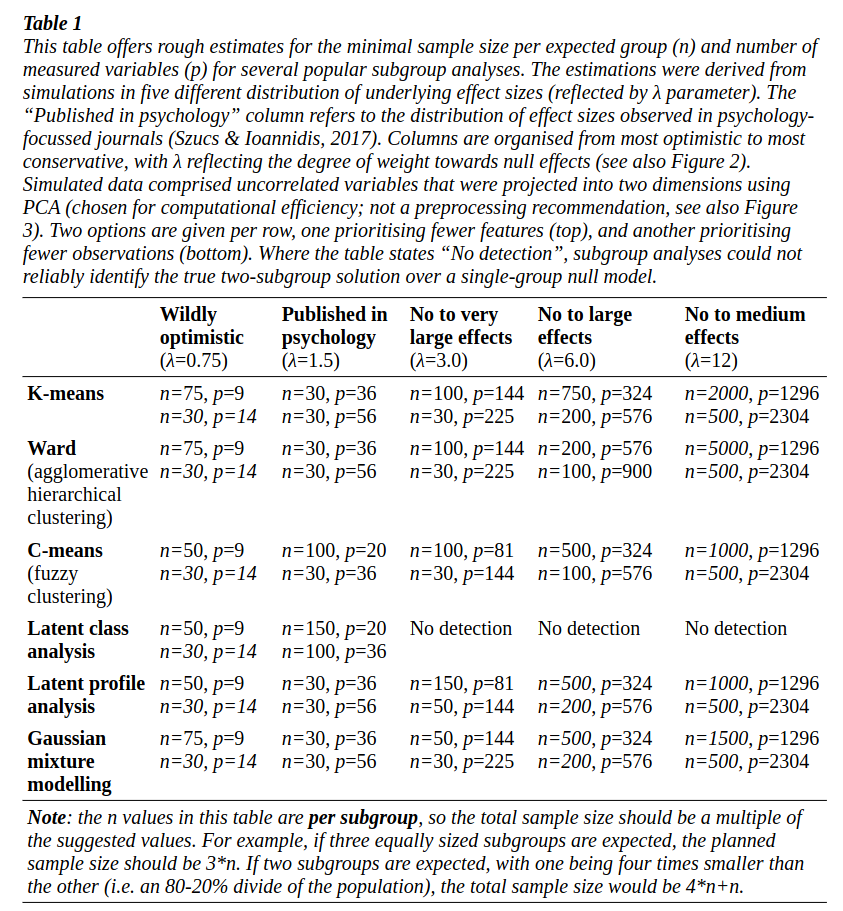# aliquote.org

### Unidimensionality in Rasch Models: Efficient Item Selection and Hierarchical Clustering Methods Based on Marginal Estimates (https://arxiv.org/abs/2309.00553)

Since I am no longer involved in psychometrics or this kind of stuff (even on Cross Validated), I rarely read this kind of papers nowadays. Selecting misfitting items on a scale has a long history, especially for Rasch modellers. This paper exposes a sort of clustering method that allows to flag item, based on the variance of a mixing distribution, that do not belong with a set of items sharing a commin trait.

### Optimal Scaling transformations to model non-linear relations in GLMs with ordered and unordered predictors (https://arxiv.org/abs/2309.00419)

Still a memory of my previous job where I used to use optimal scaling a lot, but in the context of projection methods in multivariate exploratory data analysis. Here, Meulman and collaborators consider optimal scaling as an alterantive framework to GAM to allow non linearity in discrete predictors. In particular, this alleviares the need for dummy coded variable levels, via quantization with monotonicity constraints, which facilitates the interpretation of the resulting output. There will probably an R package available at some point. See also ROS Regression: Integrating Regularization with Optimal Scaling Regression.

### An introduction to graph theory (https://arxiv.org/abs/2308.04512)

A complete course on graph theory, including network flows, for graduate students. It is rather extensive (422 pp.) and there are a lot of illustrations.

### Permutation testing in high-dimensional linear models: an empirical investigation (https://arxiv.org/abs/2001.01466)

This is an extension to the permutation testing framework to the case where the number of predictors exceeds the sample size. In classical settings ($p < n$), the startegy amounts to compute the test statistics under random permutation of the residuals.1 When $n \ll p$, however, regularization methos like the elastic net appoach msut be used. The authors recall that for minimizing prediction error, ridge regression is often preferrable to Lasso, principal components regression, variable subset selection and partial least squares. Moreover, ridge regression is close to the Freedman-Lane approach, which is based on semi-partial correlations. The authors finally suggest to use double residualization, which is inspired by the Kennedy method, which residualizes both $Y$ and $X$ and proceeds to permute the $Y$-residuals, 2 but in this paper the authors replace the least squares regression by ridge regression.

### Tutorial: a priori estimation of sample size, effect size, and statistical power for cluster analysis, latent class analysis, and multivariate mixture models (https://arxiv.org/abs/2309.00866)

This is a short review of power analysis for k-means, Ward agglomerative hierarchical clustering, c-means fuzzy clustering, latent class analysis, latent profile analysis, and Gaussian mixture modelling. Results based on simulated datasets are summarized in Table 1 of the paper, reproduced below.### Supervised dimensionality reduction for multiple imputation by chained equations (https://arxiv.org/abs/2309.01608)

In this paper, the authors discuss the use of principal component, principal covariates, and partial least squares regression, instead of unsupervised PCA, with MICE. Results show that supervised appraoches perform better, and that supervised principal component regression has smaller bias and better confidence interval coverage for a wider range of retained components, independent of the number of latent variables.

♪ Bauhaus • Stigmata Martyr

1. Freedman, D. and Lane, D. A nonstochastic interpretation of reported significance levels. Journal of Business & Economic Statistics, 1(4):292–298, 1983. ↩︎

2. Kennedy, F. E. Randomization tests in econometrics. Journal of Business & Economic Statistics, 13 (1):85–94, 1995. ↩︎